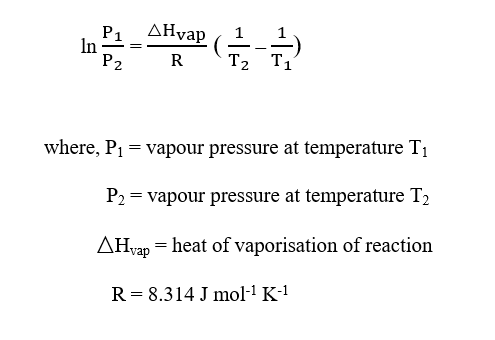# Two students measure the vapor pressure of the same unknown organic liquid and compare results. The first student measured a vapor pressure of 630.4 torr at 191 °C, while the second student measured a vapor pressure of 0.5570 atm at 160 °C. What is the ΔH°vap, in kilojoules per mole, for this substance?

Question
1 views

Two students measure the vapor pressure of the same unknown organic liquid and compare results. The first student measured a vapor pressure of 630.4 torr at 191 °C, while the second student measured a vapor pressure of 0.5570 atm at 160 °C. What is the ΔH°vap, in kilojoules per mole, for this substance?

check_circle

Step 1

The Clausius-Clapeyron equation is given as:Step 2

Given,

Vapour pressure, P1 = 630.4 torr

Vapour pressure, P2 = 0.5570 atm = (0.5570 × 760) torr = 423.32 torr

Temperature, T1 = 191 oC = (273 + 191) K = 464 K

Temperature, T2 = 160 oC = (273 + 160) K = 433 K

Therefore, the value of △Hvap can be calculated using the Clausius-Clapeyron equation a...

### Want to see the full answer?

See Solution

#### Want to see this answer and more?

Solutions are written by subject experts who are available 24/7. Questions are typically answered within 1 hour.*

See Solution
*Response times may vary by subject and question.
Tagged in

### Chemistry